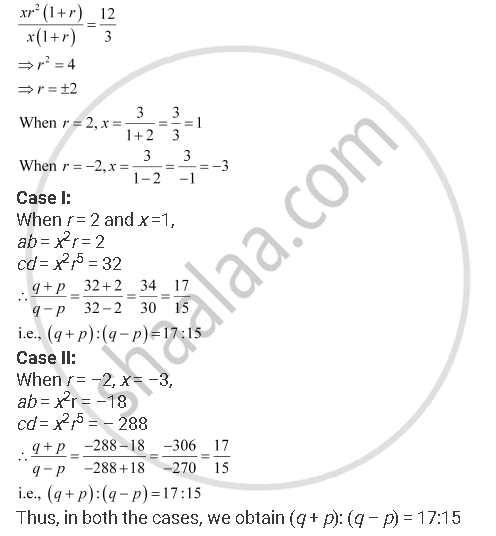CBSE (Arts) Class 11CBSE
Share

# If A And B Are the Roots Of Are Roots Of X2 – 3x + P = 0 , and C, D Are Roots of X2 – 12x + Q = 0, Where A, B, C, D, Form a G.P. Prove that (Q + P): (Q – P) = 17:15. - CBSE (Arts) Class 11 - Mathematics

ConceptGeometric Progression (G. P.)

#### Question

If a and are the roots of are roots of x2 – 3x + p = 0 , and c, d are roots of x2 – 12x + q = 0, where a, b, cd, form a G.P. Prove that (q + p): (q – p) = 17:15.

#### Solution

It is given that a and b are the roots of x– 3p = 0

∴ a + b = 3 and ab = p … (1)

Also, c and d are the roots of  x2 – 12x + q = 0

d = 12 and cd = q … (2)

It is given that abcd are in G.P.

Let xb = xrc = xr2d = xr3

From (1) and (2), we obtain

x + xr = 3

⇒ x (1 + r) = 3

xr2 + xr3 =12

⇒ xr(1 + r) = 12

On dividing, we obtainIs there an error in this question or solution?

#### APPEARS IN

NCERT Solution for Mathematics Textbook for Class 11 (2018 to Current)
Chapter 9: Sequences and Series
Q: 18 | Page no. 199

#### Video TutorialsVIEW ALL 

Solution If A And B Are the Roots Of Are Roots Of X2 – 3x + P = 0 , and C, D Are Roots of X2 – 12x + Q = 0, Where A, B, C, D, Form a G.P. Prove that (Q + P): (Q – P) = 17:15. Concept: Geometric Progression (G. P.).
S